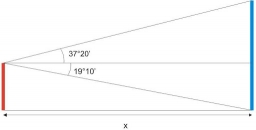# The tower

The observer sees the base of the tower 96 meters high at a depth of 30 degrees and 10 minutes and the top of the tower at a depth of 20 degrees and 50 minutes. How high is the observer above the horizontal plane on which the tower stands?

Correct result:

y2 =  37.983 m

#### Solution:

$h=96 \ \text{m} \ \\ A=30+10/60=\dfrac{ 181 }{ 6 } \doteq 30.1667 \ ^\circ \ \\ B=20+50/60=\dfrac{ 125 }{ 6 } \doteq 20.8333 \ ^\circ \ \\ \ \\ h=y_{1}+y_{2} \ \\ \tan A=y_{1}/x \ \\ \tan B=y_{2}/x \ \\ \ \\ \tan A/\tan B=y_{1}/y_{2} \ \\ \tan A/\tan B=(h-y_{2})/y_{2} \ \\ \ \\ t=\tan A ^\circ /\tan B ^\circ =\tan 30.1666666667^\circ \ /\tan 30.1666666667^\circ \ =0.581235/0.581235=1.52744 \ \\ \ \\ t=(h-y_{2})/y_{2} \ \\ y_{2} \cdot \ t=h-y_{2} \ \\ \ \\ y_{2}(t+1)=h \ \\ \ \\ y_{2}=h / (t+1)=96 / (1.5274+1) \doteq 37.9832 \doteq 37.983 \ \text{m} \ \\ \ \\ \text{ Correctness test: } \ \\ y_{1}=h - y_{2}=96 - 37.9832 \doteq 58.0168 \ \text{m} \ \\ x=y_{1} / \tan A ^\circ =y_{1} / \tan 30.1666666667^\circ \ =58.0168317089 / \tan 30.1666666667^\circ \ =58.0168317089 / 0.581235=99.816$Our examples were largely sent or created by pupils and students themselves. Therefore, we would be pleased if you could send us any errors you found, spelling mistakes, or rephasing the example. Thank you!

Leave us a comment of this math problem and its solution (i.e. if it is still somewhat unclear...):Be the first to comment!Tips to related online calculators
Check out our ratio calculator.
Do you have a system of equations and looking for calculator system of linear equations?
Most natural application of trigonometry and trigonometric functions is a calculation of the triangles. Common and less common calculations of different types of triangles offers our triangle calculator. Word trigonometry comes from Greek and literally means triangle calculation.

#### You need to know the following knowledge to solve this word math problem:

We encourage you to watch this tutorial video on this math problem:

## Next similar math problems:

• Circular poolThe base of the pool is a circle with a radius r = 10 m, excluding a circular segment that determines the chord length 10 meters. The pool depth is h = 2m. How many hectoliters of water can fit into the pool?
• The trapeziumThe trapezium is formed by cutting the top of the right-angled isosceles triangle. The base of the trapezium is 10 cm and the top is 5 cm. Find the area of trapezium.Show that the quadrilateral with vertices P1(0,1), P2(4,2) P3(3,6) P4(-5,4) has two right triangles.
• Ratio of sidesCalculate the area of a circle that has the same circumference as the circumference of the rectangle inscribed with a circle with a radius of r 9 cm so that its sides are in ratio 2 to 7.
• Annular areaThe square with side a = 1 is inscribed and circumscribed by circles. Find the annular area.
• Circular segmentCalculate the area S of the circular segment and the length of the circular arc l. The height of the circular segment is 2 cm and the angle α = 60°. Help formula: S = 1/2 r2. (Β-sinβ)
• DecagonCalculate the area and circumference of the regular decagon when its radius of a circle circumscribing is R = 1m
• NonagonCalculate the area and perimeter of a regular nonagon if its radius of inscribed circle is r = 10cm
• 30-gonAt a regular 30-gon the radius of the inscribed circle is 15cm. Find the "a" side size, circle radius "R", circumference, and content area.
• WasteHow many percents are waste from a circular plate with a radius of 1 m from which we cut a square with the highest area?
• Quarter circleWhat is the radius of a circle inscribed in the quarter circle with a radius of 100 cm?
• Circular ringSquare with area 16 centimeters square are inscribed circle k1 and described circle k2. Calculate the area of circular ring, which circles k1, k2 form.
• FlakesA circle was described on the square, and a semicircle above each side of the square was described. This created 4 "flakes". Which is bigger: the content of the central square or the content of four chips?
• Company logoThe company logo consists of a blue circle with a radius of 4 cm, which is an inscribed white square. What is the area of the blue part of the logo?
• Area of a rectangleCalculate the area of a rectangle with a diagonal of u = 12.5cm and a width of b = 3.5cm. Use the Pythagorean theorem.
• BillboardRectangular billboard is 2.5 m long with a diagonal 2.8 m long. Calculate the perimeter and the content area of the billboard.
• SquareCalculate the perimeter and the area of square with a diagonal length 30 cm.